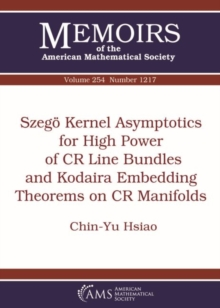# Szego Kernel Asymptotics for High Power of CR Line Bundles and Kodaira Embedding Theorems on CR Manifolds Paperback / softback

## Part of the Memoirs of the American Mathematical Society series

#### Description

Let $X$ be an abstract not necessarily compact orientable CR manifold of dimension $2n-1$, $n\geqslant 2$, and let $L^k$ be the $k$-th tensor power of a CR complex line bundle $L$ over $X$.

Given $q\in \{0,1,\ldots ,n-1\}$, let $\Box ^{(q)}_{b,k}$ be the Gaffney extension of Kohn Laplacian for $(0,q)$ forms with values in $L^k$.

For $\lambda \geq 0$, let $\Pi ^{(q)}_{k,\leq \lambda} :=E((-\infty ,\lambda ])$, where $E$ denotes the spectral measure of $\Box ^{(q)}_{b,k}$.

In this work, the author proves that $\Pi ^{(q)}_{k,\leq k^{-N_0}}F^*_k$, $F_k\Pi ^{(q)}_{k,\leq k^{-N_0}}F^*_k$, $N_0\geq 1$, admit asymptotic expansions with respect to $k$ on the non-degenerate part of the characteristic manifold of $\Box ^{(q)}_{b,k}$, where $F_k$ is some kind of microlocal cut-off function.

Moreover, we show that $F_k\Pi ^{(q)}_{k,\leq 0}F^*_k$ admits a full asymptotic expansion with respect to $k$ if $\Box ^{(q)}_{b,k}$ has small spectral gap property with respect to $F_k$ and $\Pi^{(q)}_{k,\leq 0}$ is $k$-negligible away the diagonal with respect to $F_k$.

By using these asymptotics, the authors establish almost Kodaira embedding theorems on CR manifolds and Kodaira embedding theorems on CR manifolds with transversal CR $S^1$ action.

#### Information

• Format: Paperback / softback
• Pages: 140 pages
• Publisher: American Mathematical Society
• Publication Date:
• Category: Algebra
• ISBN: 9781470441012

£75.50

£74.19

on all orders

###### Pick up orders

from local bookshops

£77.95

£77.35

£77.95

£77.35

£77.95

£77.35

£77.95

£77.35